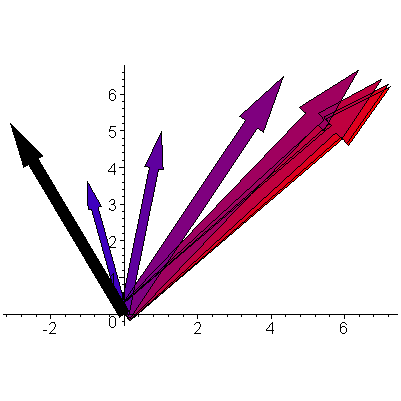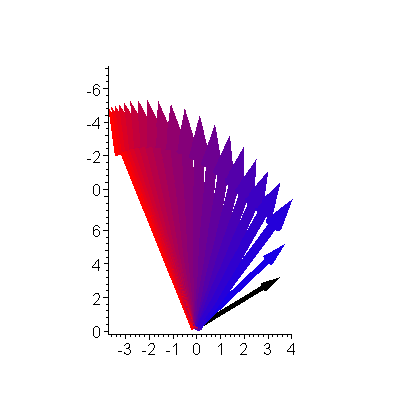# Introduction

There are numerous numerical techniques for finding eigenvalues and eigenvectors. In this topic, we will look at an elegant method of finding the eigenvalue of a matrix which has all positive eigenvalues. This is our first example of a numerical technique which is based on iteration.

# Background

Useful background for this topic includes:

# References

• Bradie, Section 4.1, The Power Method, p.265.
• Mathews, Section 11.2, The Power Method, p.598.
• James [AMEM], Section 6.5.1 The Power Method, p.446.

# Interactive Maplet

A Power Method Demonstration

# Theory

Suppose v is an eigenvector of a diagonalizable n × n matrix M. Then Mv = λv for some value λ. Thus, given any real number c > 0, we may calculate:

If |λ/c| < 1 then the limit as k → ∞ is the zero vector 0, and if λ = c, this limit is v.

Thus, given an n × n matrix M with real eigenvalues, let λ1λ2 ≥ ⋅⋅⋅ ≥ λn be the eigenvalues ordered from largest to smallest and let v1, ..., vn be the corresponding eigenvectors. Therefore, a random vector u may be written as:

u = u1 v1 + u2 v2 + ⋅⋅⋅ + un vn

where, if the vector is truly random, u1 ≠ 0.

Multiplying by M1 yields:

(M1) u = (u1/λ1) Mv1 + (u2/λ1) Mv2 + ⋅⋅⋅ + (un/λ1) Mvn
= u1 (λ1/λ1) v1 + u2 (λ2/λ1) v2 + ⋅⋅⋅ + un (λn/λ1) vn

and hence, multiplying u by (M1)k yields:

(M1)k u = u1 (λ1/λ1)k v1 + u2 (λ2/λ1)k v2 + ⋅⋅⋅ + un (λn/λ1)k vn

The first ratio is 1 and (1)k = 1 while all other ratios are less than one, and therefore all other ratios go to zero, and thus

(M1)k uu1 v1 as k → ∞

Thus, if this sequence approaches the maximum eigenvector v1. Now, we use the property of the norm that:

||α v1||2 = |α| ||v1||2

for any scalar α to find the largest eigenvalue as follows:

Because we assumed that λ1 > 0, we simply calculate λ1 = ||M(u1v1)||2/||u1v1||2.

The howto describes the numerical technique for finding this largest eigenvalue and its corresponding eigenvector.

# Problem

Given a matrix M with real coefficients, find the maximum eigenvalue.

# Assumptions

We assume that the largest eigenvalue is real and positive and that the matrix is diagonalizable.

# Tools

We will use basic linear algebra and iteration.

# Initial Requirements

We begin with a random unit vector v0. There are certain vectors for which this method will not converge to the largest eigenvalue, however, the probability of choosing such a vector randomly is zero.

# Iteration Process

Given the vector vk, calculate vk + 1 = Mvk/||vk||2 where ||vk||2 is the Euclidean norm of vk.

# Halting Conditions

There are two conditions which may cause the iteration process to halt:

1. We halt if the difference between successive vectors is sufficiently small, that is, ||vk + 1 - vk||2 < εstep,
2. If we have iterated some maximum number of times, say N, and have not met Condition 1, we halt and indicate that a solution was not found.

If we halt due to Condition 1, we state that ||vk + 1||2 is our approximation to the eigenvalue and vk + 1 is our approximation to the corresponding eigenvector.

If we halt due to Condition 2, then either the largest eigenvalue is complex or the two largest eigenvalues are very close in absolute value.

# Error Analysis

You will note that with every iteration, we divided the vector by its absolute value. While in theory, we could simply continue multiplying v0 by higher and higher powers of M and look at the relative norms, because we are using the double format to store numbers, the growth in the magnitude will be exponential, and thus we will very quickly either overflow or underflow.

For example, if the resistors in a circuit are on the order of 1 kΩ, then a matrix of resistances may have a norm on the order 1000, and therefore, with as few as 50 iterations, an overflow may occur.

# Example 1

Approximate the maximum eigenvalue of the matrix with the halting value εstep = 1e-10 and N = 200.

Adapting the code on the Matlab page:

```>> format long
>> M = [8 3 -4; 3 7 2; -4 2 9];
>> v = rand( 3, 1 );
>> oldnorm = Inf;       % set old norm to infinity
>> for i=1:100
v = M * v / norm(v);
newnorm = norm(v);

if ( abs( newnorm − oldnorm ) < 1e-10 )
break;
elseif ( i == 100 )
error( 'method failed to converge' );
else
oldnorm = newnorm;
end
end
>> newnorm
newnorm = 12.5899223267361
>> i       % check to see the number of iterations, this may differ
i = 51
```

# Example 2

Show, using Matlab, that the maximum eigenvalue of the matrix [0 1; 1 -1] is φ, the golden ratio.

```>> M = [0 -1; -1 1];
>> v = rand( 2, 1 );
>> for i=1:100
vold = v;

v = M*v / norm( v );

if ( abs( norm( v ) − norm( vold ) ) < 1e-5 )
break;
elif ( i = 100 )
error( 'method failed to converge' );
end
end
>> i         % the number of iterations
i = 9
>> v         % the eigenvector
v =

-0.851283180611599
1.375989714216622

>> norm( v )           % the eigenvalue
ans = 1.61803298706242
```

# Example 3

Plot the sequence of approximations of the maximum eigenvector for the matrix

starting with the initial vector

These are shown in Figure 1. The sequence of approximations is shaded from blue to red. The last plotted red vector is quite close to the actual eigenvector of 9.54 ⋅ (0.763, 0.646)T (9.54 being the corresponding eigenvalue).Figure 1. The sequence of approximations of the maximum eigenvector with the initial vector v = (-3.1, 5.2)T in black.

# Example 4

Plot the sequence of approximations of the maximum eigenvector for the matrix

starting with the initial vector

These are shown in Figure 2. The sequence of approximations is shaded from blue to red. The last plotted red vector is quite close to the actual eigenvector of 10.1 ⋅ (0.746, 0.423, -0.515)T (10.1 being the corresponding eigenvalue).Figure 2. The sequence of approximations of the maximum eigenvector with the initial vector v = (-2.1, 3.5, 0.9)T in black.

# Question 1

Use Matlab using the technique described in this topic to find the largest eigenvalue of the matrix

with εstep = 1e-10 and N = 100 and starting with the random vector (4/5, 3/5, 0)T.

(13.7851650423974)

# Question 2

Assuming that eig returns the correct eigenvalue, what is the relative error of the approximation found in Question 1?

(4.8e-13)

# Question 3

Show what happens to this algorithm if the random vector is the kth eigenvector.

(The technique converges to the corresponding eigenvalue as opposed to the maximum eigenvalue.)

# Applications to Engineering

The maximum eigenvalue is used in finding the condition number of a matrix. The condition number indicates how good a solution found using numerical techniques can be.

# Matlab

The eig function returns the eigenvalues of a matrix, and thus, the following code works:

```>> M = rand( 5, 5 );        % create a random matrix
>> M = M' * M;              % make it positive definite
>> max( eig( M ) )          % use the built-in functions
```

The technique described in this topic is given below with εstep = 1e-5 and N = 100:

```>> v = rand( 5, 1 );
>> v = v / norm(v);     % normalize it
>> oldnorm = Inf;       % set old norm to infinity
>> for i=1:100
v = M * v;
newnorm = norm(v);

if ( abs( newnorm − oldnorm ) < 1e-5 )
break;
elseif ( i == 100 )
error( 'method failed to converge' );
else
v = v/newnorm;
oldnorm = newnorm;
end
end
newnorm
```

# Maple

The eigenvalues of a matrix may be found with the Eigenvalues command:

```M := <<-6, 12, 2.4, 0>|<-1, 2, 10.4, 1>|<3.25, 1, -1.8, 14.8>|<10.25, 0, 2, 1.2>>;
LinearAlgebra[Eigenvalues]( M );
```

For more help on either of these routines or on the LinearAlgebra package, enter:

```?LinearAlgebra
?LinearAlgebra,Eigenvalues
```